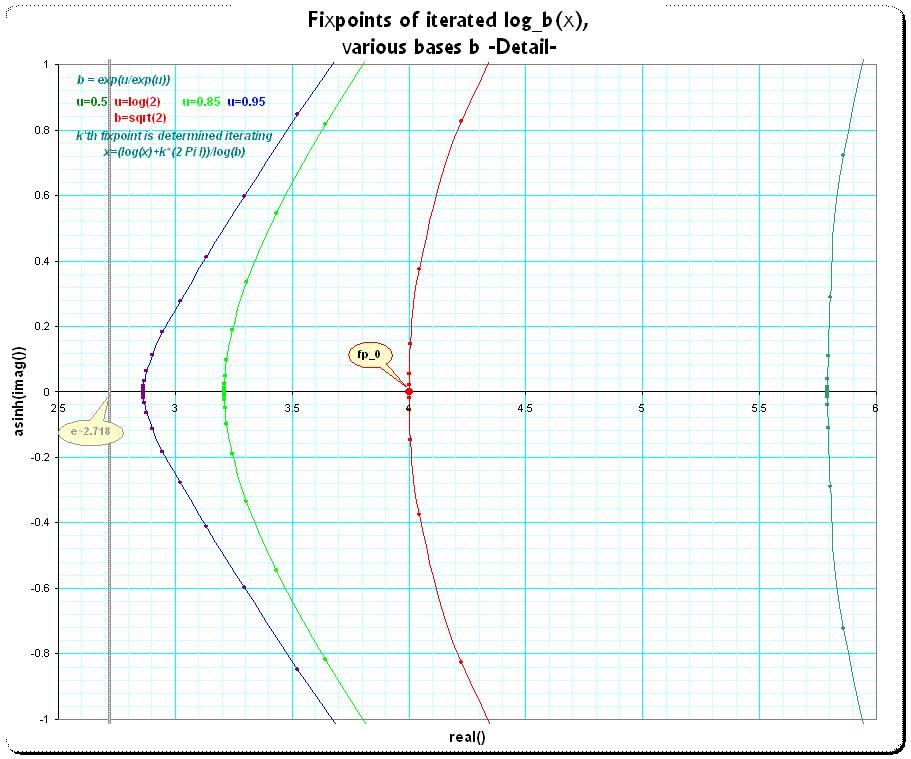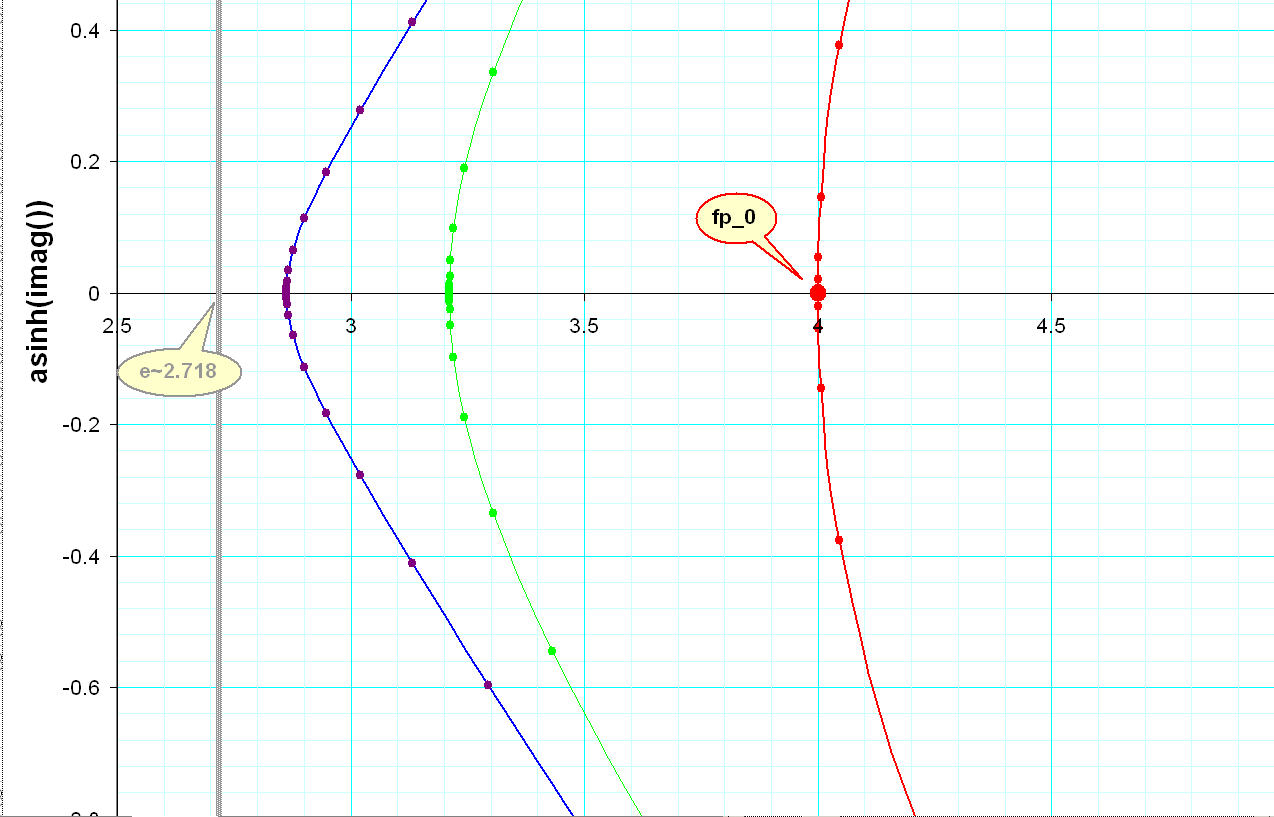[UFO] Attracting Fixpoints or attracting line? - Printable Version +- Tetration Forum (https://math.eretrandre.org/tetrationforum) +-- Forum: Tetration and Related Topics (https://math.eretrandre.org/tetrationforum/forumdisplay.php?fid=1) +--- Forum: Mathematical and General Discussion (https://math.eretrandre.org/tetrationforum/forumdisplay.php?fid=3) +--- Thread: [UFO] Attracting Fixpoints or attracting line? (/showthread.php?tid=422) Pages: 1 2 [UFO] Attracting Fixpoints or attracting line? - Gottfried - 02/22/2010 Pre-note: Sometimes I'm considering ideas, which may be a bit far out, strange or even trivial but not been resolved in a second view (well maybe they would on a third view...), but if they seem(ed) surprising and interesting enough I posted some of them here already. I think in general this is appropriate, but such sketchy things may marked as such, so the tag "UFO" for such msgs may be the most meaningful. Feel free to just ignore "UFO"-tagged msgs ... ------------------ I looked for a list of the complex fixpoints of the tetration and whether there are some more interesting regularities. So, for instance the base b=sqrt(2): let's look at the list of the fixpoints. First: as well known, there is only one attracting fixpoint a=2. Then we have the repelling fixpoint r0=4. And then the infinite list of complex fixpoints, all repelling. Ok, if we apply the iterated log instead of the iterated exponential they become attracting and the fixpoint a0 becomes repelling. How do we get the different fixpoints r0,r1,r2, ... r_k,... (with each r_k also the conjugate is a fixpoint) ? We use the k'th branch of the log, so r_k = lim_{n->inf} x = (log(x) + k*2*Pi*I ) / log(b) When I looked at the plot of that (isolated) fixpoints I asked, whether we could interpolate a line. And indeed, we can simply take any real k to get r_k = lim_{n->inf} x = (log(x) + k*2*Pi*I ) / log(b) converging! So this means, we have in fact an attracting continuous curve, and the usual fixpoints are simply that where k is integer... I've drawn a plot with a couple of bases: b = exp(u*exp(-u)) , where if u=log(2) we have our favorite one b=sqrt(2). We get the first two fixpoints r0 = 4 , r1 ~ 9.09... + i*21.5 etc; and the fixpoint a = 2, which is repelling if we use logarithmizing. The plot shows the curves for u = 0.5, u=log(2), u=0.85, u=0.95, where dots are inserted at the integer k's (marking the commonly used fixpoints). It is interesting to approach the critical base b=e^(1/e), meaning u->1 from below, however I do not yet have a good idea of the geometry of that limiting curves. Here are the original plot, the value of the imaginary part scaled by asinh() (which means nearly a log-scaling, but allows the zero- and negativ values to be rescaled) and two details near the real fixpoints to see the smoothness of the curves. GottfriedRE: [UFO] Attracting Fixpoints or attracting line? - bo198214 - 02/24/2010 Hm, interesting idea. For this line would however have a gap near the real axis? RE: [UFO] Attracting Fixpoints or attracting line? - Gottfried - 02/24/2010 (02/24/2010, 11:16 AM)bo198214 Wrote: Hm, interesting idea. For this line would however have a gap near the real axis? Mhh, possibly we find then the origin of the curves in my (very) old plots concerning the complex fixpoints of such real basesand(where the black lines show the locus of the complex numbers t, such that and b is real using that idea... Gottfried RE: [UFO] Attracting Fixpoints or attracting line? - tommy1729 - 02/27/2010 euh ... what is your intention with this attracting line ? i was thinking and thinking and thinking about how you would use that for tetration , but i dont see how. care to explain ? RE: [UFO] Attracting Fixpoints or attracting line? - Gottfried - 02/27/2010 (02/27/2010, 11:11 PM)tommy1729 Wrote: euh ... what is your intention with this attracting line ? i was thinking and thinking and thinking about how you would use that for tetration , but i dont see how. care to explain ? Actually I do not know exactly. First -We have this nasty problem, that regular iteration around different fixpoints give different fractional heights - but actually we do not know the difference, only that there occurs some wobbling and the difference vanishes at integer heights. Now we have some meaningful continuous line between fixpoints - maybe that gives another key for some functional description of that differences. Second - range of convergence is an important topic. If we use the powerseries for iteration and a point is too far from the fixpoint, we get divergence. So we have the option to use another fixpoint. Say, base=sqrt(2). Then iterating x0=5 using the schröder-function to implement fractional iteration gives divergence, when the series is developed around the fixpoint 2. But that series developed around the other fixpoint 4 gives nicely converging sums. Now the fixpoints are separated; if we can use some arbitrary value from the "fixed-line" for the construction of the powerseries, say the complex coordinate nearest to x (x=5 in the example), then -perhaps- we can improve convergence - don't know yet. The whole idea is just an "UFO" - unidentified floating observation ;-) ; let's see, what we can do with it... Gottfried RE: [UFO] Attracting Fixpoints or attracting line? - tommy1729 - 02/28/2010 (02/27/2010, 11:53 PM)Gottfried Wrote: Second - range of convergence is an important topic. If we use the powerseries for iteration and a point is too far from the fixpoint, we get divergence. So we have the option to use another fixpoint. Say, base=sqrt(2). Then iterating x0=5 using the schröder-function to implement fractional iteration gives divergence, when the series is developed around the fixpoint 2. But that series developed around the other fixpoint 4 gives nicely converging sums. Now the fixpoints are separated; if we can use some arbitrary value from the "fixed-line" for the construction of the powerseries, say the complex coordinate nearest to x (x=5 in the example), then -perhaps- we can improve convergence - don't know yet. The whole idea is just an "UFO" - unidentified floating observation ;-) ; let's see, what we can do with it... Gottfried i think the range of convergence problem can be solved by using formal power series and then using mittag-leffler expansion for the ' actual numerical computation '. regards tommy1729 RE: [UFO] Attracting Fixpoints or attracting line? - Gottfried - 04/02/2010 (02/24/2010, 11:16 AM)bo198214 Wrote: Hm, interesting idea. For this line would however have a gap near the real axis? I show a slightly modified type of plot. Here I use b=exp(1). I found, that the complex fixpoints t_k can be parametrized in terms of their real part. Denote a fixpoint t_k = a + b*i, then I can select some a and compute the required b. Then I can also compute the number k by taking t_k- log(t_k). It is more difficult to compute t_k by choosing k: we must iterate x = log(x) + 2PiI*k many times as usual, however the only possibility to find the fixpoints for exactly integer k. In the plot the blue lines are the locus of the fixpoints assuming continuous real k. The fixpoints at integer k are marked with big red points; the "fixpoint" at k/2 is marked by a star. The blue lines are naturally continuous *below* real(t_0)~0.3181... however, selecting any real k we cannot find that points. In fact, if we compute such a point using, for instance, a=0.1, then we get some meaningful b and the according k, but if we resinsert that k to compute the associated fixpoint we end in the curve with real parts *above* real(t_0). So I mared that "inaccessibl area" lefthand in the plot with a grey shade. The orange lines are the computed (continuous) indexes k; their y-scale is the orange scale on the right side of the plot (the line has abscissa "1" at the fixpoint t_k at k=1 and abscissa "0" at fixpoint t_k at k=0 ) -------------------------------------- [update] I actually do not yet know how this can be useful - I've not yet used such a fixpoint-shift for the recentering of the exp-function with base e seriously, it was always somehow messy.... [/update] Here is the plot: RE: [UFO] Attracting Fixpoints or attracting line? - tommy1729 - 04/07/2010 best pic of the year till nowbut i dont know how to " quote " it , i can only quote text when i try ? the returning crazy idea has come to my mind again ; extending the complex numbers to arrive at fixpoints for all parameters , when there are no complex fixpoints. its a diseasejust like tetration :p regards tommy1729 RE: [UFO] Attracting Fixpoints or attracting line? - tommy1729 - 04/08/2010 (04/02/2010, 12:40 PM)Gottfried Wrote: (02/24/2010, 11:16 AM)bo198214 Wrote: Hm, interesting idea. For this line would however have a gap near the real axis? I show a slightly modified type of plot. Here I use b=exp(1). I found, that the complex fixpoints t_k can be parametrized in terms of their real part. Denote a fixpoint t_k = a + b*i, then I can select some a and compute the required b. Then I can also compute the number k by taking t_k- log(t_k). It is more difficult to compute t_k by choosing k: we must iterate x = log(x) + 2PiI*k many times as usual, however the only possibility to find the fixpoints for exactly integer k. In the plot the blue lines are the locus of the fixpoints assuming continuous real k. The fixpoints at integer k are marked with big red points; the "fixpoint" at k/2 is marked by a star. The blue lines are naturally continuous *below* real(t_0)~0.3181... however, selecting any real k we cannot find that points. In fact, if we compute such a point using, for instance, a=0.1, then we get some meaningful b and the according k, but if we resinsert that k to compute the associated fixpoint we end in the curve with real parts *above* real(t_0). So I mared that "inaccessibl area" lefthand in the plot with a grey shade. The orange lines are the computed (continuous) indexes k; their y-scale is the orange scale on the right side of the plot (the line has abscissa "1" at the fixpoint t_k at k=1 and abscissa "0" at fixpoint t_k at k=0 ) -------------------------------------- [update] I actually do not yet know how this can be useful - I've not yet used such a fixpoint-shift for the recentering of the exp-function with base e seriously, it was always somehow messy.... [/update] Here is the plot: uhm ... your plot gives the fixed points of exp( t_k + k2pi i ) = t_k but should that not be periodic with k ? since exp( x + k i ) is periodic with real k ? im a bit confused now ... ah i see , you only plotted from k in (-1,1) so that pic IS the period ...RE: [UFO] Attracting Fixpoints or attracting line? - bo198214 - 04/09/2010 (04/08/2010, 10:03 PM)tommy1729 Wrote: your plot gives the fixed points of exp( t_k + k2pi i ) = t_k but should that not be periodic with k ? since exp( x + k i ) is periodic with real k ? im a bit confused now ... No, the fixed points of exp, i.e. the points with exp(z)=z, can be obtained by considering , i.e. the fixed points of the branches of the logarithm. All the non-real fixed points of exp are repelling, i.e. |exp(z)|>1, thats why these fixed points are attracting for the logarithm and can be obtained by iterating the corresponding branch. Gottfried's question was now what happens if we choose non-integer ; and found that the corresponding "fixed points" lie smoothly on a line between the proper fixed points of .